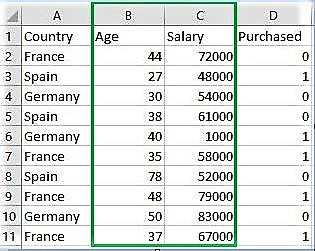Related Articles
sklearn.Binarizer() in Python
• Last Updated : 10 May, 2019

sklearn.preprocessing.Binarizer() is a method which belongs to preprocessing module. It plays a key role in the discretization of continuous feature values.

Example #1:
A continuous data of pixels values of an 8-bit grayscale image have values ranging between 0 (black) and 255 (white) and one needs it to be black and white. So, using `Binarizer()` one can set a threshold converting pixel values from 0 – 127 to 0 and 128 – 255 as 1.

Example #2:
One has a machine record having “Success Percentage” as a feature. These values are continuous ranging from 10% to 99% but a researcher simply wants to use this data for prediction of pass or fail status for the machine based on other given parameters.

Syntax :

`sklearn.preprocessing.Binarizer(threshold, copy)`

Parameters :

threshold :[float, optional] Values less than or equal to threshold is mapped to 0, else to 1. By default threshold value is 0.0.
copy :[boolean, optional] If set to False, it avoids a copy. By default it is True.

Return :

`Binarized Feature values`Below is the Python code explaning sklearn.Binarizer()

 `# Python code explaining how``# to Binarize feature values``  ` `""" PART 1``    ``Importing Libraries """``  ` `import` `numpy as np``import` `matplotlib.pyplot as plt``import` `pandas as pd`` ` `# Sklearn library ``from` `sklearn ``import` `preprocessing`` ` `""" PART 2``    ``Importing Data """``  ` `data_set ``=` `pd.read_csv(``        ``'C:\\Users\\dell\\Desktop\\Data_for_Feature_Scaling.csv'``)``data_set.head()`` ` `# here Features - Age and Salary columns ``# are taken using slicing``# to binarize values``age ``=` `data_set.iloc[:, ``1``].values``salary ``=` `data_set.iloc[:, ``2``].values``print` `(``"\nOriginal age data values : \n"``,  age)``print` `(``"\nOriginal salary data values : \n"``,  salary)`` ` `""" PART 4``    ``Binarizing values """`` ` `from` `sklearn.preprocessing ``import` `Binarizer`` ` `x ``=` `age``x ``=` `x.reshape(``1``, ``-``1``)``y ``=` `salary``y ``=` `y.reshape(``1``, ``-``1``)`` ` `# For age, let threshold be 35``# For salary, let threshold be 61000``binarizer_1 ``=` `Binarizer(``35``)``binarizer_2 ``=` `Binarizer(``61000``)`` ` `# Transformed feature``print` `(``"\nBinarized age : \n"``, binarizer_1.fit_transform(x))`` ` `print` `(``"\nBinarized salary : \n"``, binarizer_2.fit_transform(y))`

Output :

```   Country  Age  Salary  Purchased
0   France   44   72000          0
1    Spain   27   48000          1
2  Germany   30   54000          0
3    Spain   38   61000          0
4  Germany   40    1000          1

Original age data values :
[44 27 30 38 40 35 78 48 50 37]

Original salary data values :
[72000 48000 54000 61000  1000 58000 52000 79000 83000 67000]

Binarized age :
[[1 0 0 1 1 0 1 1 1 1]]

Binarized salary :
[[1 0 0 0 0 0 0 1 1 1]]
```

Attention reader! Don’t stop learning now. Get hold of all the important DSA concepts with the DSA Self Paced Course at a student-friendly price and become industry ready.  To complete your preparation from learning a language to DS Algo and many more,  please refer Complete Interview Preparation Course.

In case you wish to attend live classes with industry experts, please refer Geeks Classes Live and Geeks Classes Live USA

My Personal Notes arrow_drop_up